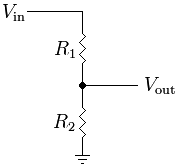## Voltage Divider Calculator

 Vin: Volts Ra: Ohms Rb: Ohms Vout: Volts

The Voltage Divider Calculator to find the Vin, Ra, Rb, Vout, when you enter three values then will get the last one.

### Voltage Divider Formula:

Vout = R2/(R1+R2) × Vin

Where Vin is Input Voltage, Vout is Output VoltageFor example, when Vin is 4 Volts, Rb is 2 Ohms, Vout is 1 Volts, then the Ra will be 6 Ohms.

Thinkcalculator.com provides you helpful and handy calculator resources.